## Example Questions

### Example Question #202 : Algebra

Each of the following is equivalent to

xy/z * (5(x + y))  EXCEPT:

xy(5x + 5y)/z

xy(5y + 5x)/z

5x²y + 5xy²/z

5x² + y²/z

5x² + y²/z

Explanation:

Choice a is equivalent because we can say that technically we are multiplying two fractions together: (xy)/z and (5(x + y))/1.  We multiply the numerators together and the denominators together and end up with xy (5x + 5y)/z.  xy (5y + 5x)/z is also equivalent because it is only simplifying what is inside the parentheses and switching the order- the commutative property tells us this is still the same expression.  5x²y + 5xy²/z is equivalent as it is just a simplified version when the numerators are multiplied out.  Choice 5x² + y²/z is not equivalent because it does not account for all the variables that were in the given expression and it does not use FOIL correctly.

### Example Question #3 : Inequalities

Let S be the set of numbers that contains all of values of x such that 2x + 4 < 8. Let T contain all of the values of x such that -2x +3 < 8. What is the sum of all of the integer values that belong to the intersection of S and T?

2

-2

0

-3

-7

-2

Explanation:

First, we need to find all of the values that are in the set S, and then we need to find the values in T. Once we do this, we must find the numbers in the intersection of S and T, which means we must find the values contained in BOTH sets S and T.

S contains all of the values of x such that 2x + 4 < 8. We need to solve this inequality.

2x + 4 < 8

Subtract 4 from both sides.

2x < 4

Divide by 2.

x < 2

Thus, S contains all of the values of x that are less than (but not equal to) 2.

Now, we need to do the same thing to find the values contained in T.

-2x + 3 < 8

Subtract 3 from both sides.

-2x < 5

Divide both sides by -2. Remember, when multiplying or dividing an inequality by a negative number, we must switch the sign.

x > -5/2

Therefore, T contains all of the values of x that are greater than -5/2, or -2.5.

Next, we must find the values that are contained in both S and T. In order to be in both sets, these numbers must be less than 2, but also greater than -2.5. Thus, the intersection of S and T consists of all numbers between -2.5 and 2.

The question asks us to find the sum of the integers in the intersection of S and T. This means we must find all of the integers between -2.5 and 2.

The integers between -2.5 and 2 are the following: -2, -1, 0, and 1. We cannot include 2, because the values in S are LESS than but not equal to 2.

Lastly, we add up the values -2, -1, 0, and 1. The sum of these is -2.

### Example Question #1 : Inequalities

What is the solution set of the inequality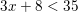?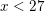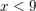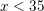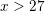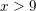Explanation:

We simplify this inequality similarly to how we would simplify an equation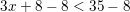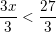Thus### Example Question #7 : Inequalities

What is a solution set of the inequality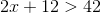?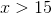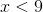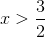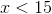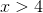Explanation:

In order to find the solution set, we solveas we would an equation: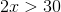Therefore, the solution set is any value of.

### Example Question #41 : Inequalities

Which of the following could be a value of, given the following inequality?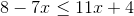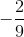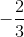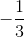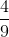Explanation:

The inequality that is presented in the problem is:Start by moving your variables to one side of the inequality and all other numbers to the other side: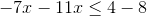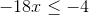Divide both sides of the equation by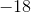. Remember to flip the direction of the inequality's sign since you are dividing by a negative number!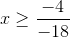Reduce: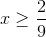The only answer choice with a value greater than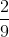is.

### Example Question #42 : Inequalities

If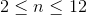and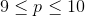, which of the following gives the set of possible values of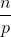?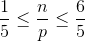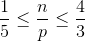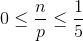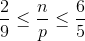Explanation:

To get the lowest value, you need the lowest numerator and the highest denominator.  That would be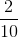or reduced to be.  For the highest value, you need the highest numerator and the lowest denominator.  That would be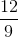or.

### Example Question #43 : Inequalities

Give the solution set of this inequality: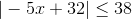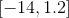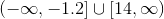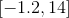The inequality has no solution.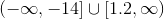Explanation:

The inequalitycan be rewritten as the three-part inequality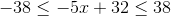Isolate thein the middle expression by performing the same operations in all three expressions. Subtract 32 from each expression: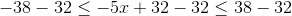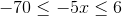Divide each expression by, switching the direction of the inequality symbols: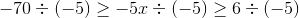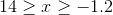This can be rewritten in interval notation as.

### Example Question #41 : Inequalities

Give the solution set of this inequality: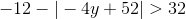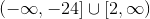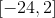The inequality has no solution.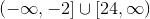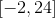The inequality has no solution.

Explanation:

In an absolute value inequality, the absolute value expression must be isolated first, as follows: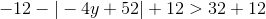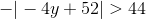Multiplying both sides by, and switching the inequality symbol due to multiplication by a negative number: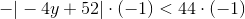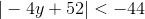We do not need to go further. An absolute value expression must always be greater than or equal to 0; it is impossible for the expression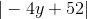to be less than any negative number. The inequality has no solution.